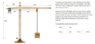# Forces acting on the base of a crane

• mathewgreenwood91

#### mathewgreenwood91

Homework Statement
Forces acting on the base of a crane
Relevant Equations
Sum of moments = 0
Sum of Forces (y) = 0
Sume of Forces (x) = 0
I am attempting the work out the forces acting on the base of a crane.

I am yet to come across forces at the base of a crane and am confused as to how to work out the reactionary/moment forces.

Any help would be greatly appreciated.

#### Attachments

•ForcesA.JPG
40.4 KB · Views: 1,150am attempting the work out the forces acting on the base of a crane
What have you got so far ? Just saying dunno, confused, doesn't count in PF

Understood sorry for not including enough details.

First I assumed that RAx = 0 as there are no forces acting in the x directions.

To work out RAy I calculated all the downward forces in Newtons, including the counterweight of 14 tonne, the concrete panel of 700kg and the self weight of the horizontal section of the crane. I am confused whether to include the self-weight of the vertical section of the crane as a downwards force acting on point A. Knowing all these forces I know the sum of Ry = 0 so I should be able to work out RAy.

My second point of confusion relates to the MA. I know the sum of moments = 0, however I have only ever done this about a point on a horizontal beam - now I am asked to work it out about point A which has the vertical beam and the perpendicular horizontal beam. I understand moments about a point = F x d but am confused by the perpendicular aspect of this problem.

First I assumed that RAx = 0 as there are no forces acting in the x directions.
There are no forces in the x-direction, so RAx=0. Not an assumption but a conclusionTo work out RAy I calculated all the downward forces in Newtons, including the counterweight of 14 tonne, the concrete panel of 7000 kg and the self weight of the horizontal section of the crane. I am confused whether to include the self-weight of the vertical section of the crane as a downwards force acting on point A. Knowing all these forces I know the sum of Ry = 0 so I should be able to work out RAy.
Why the confusion ? If you stand still on a scale that means the scale pushes you up with a reaction force equal and opposite to ##m\vec g##

My second point of confusion relates to the MA. I know the sum of moments = 0, however I have only ever done this about a point on a horizontal beam - now I am asked to work it out about point A which has the vertical beam and the perpendicular horizontal beam. I understand moments about a point = F x d but am confused by the perpendicular aspect of this problem.
Yes, moment ##\equiv## Force ##\times## perpendicular distance.
(##\ \vec \tau = \vec r \times\vec F \ ##)
Complete the list and post:
-14 m ##\times## 14000 kg * ## \vec g\ ##= .. N.m
28 m ##\times## 7000 kg * ## \vec g \ ##= .. N.m
...

Thanks for following up.

Ok, So RAy should be worked out as the following:
RAy = (14,000kg x 9.81) + (250kg/m x 68m x 9.81) + (7000kg x 9.81) + (270kg/m x 60m x 9.81)
i.e. all the downward forces including the self weight of the vertical section of the crane.

-14 m × 14000 kg * g = -1922.76 kNm
28 m × 7000 kg * g = 1,922.76 kNm
16 m x 250 kg/m x 68m * g = 2,688.32 kNm
So MA should be -2,688.32 kNm?

Is this correct or am I missing something?

16 m x 250 kg/m x 68m * g = 2,688.32 kNm
How did you calculate that 16m?

I am assuming that the self weight of the horizontal section of the crane is acting at the centre point. 16m is the distance from the centre of the cranes horizontal section to the intersection point of the crane (point I am calculating moment from)

I am assuming that the self weight of the horizontal section of the crane is acting at the centre point. 16m is the distance from the centre of the cranes horizontal section to the intersection point of the crane (point I am calculating moment from)
Yes I understand that is what you intended to calculate; I am asking for the details of the calculation of 16m.

Total distance of horizontal section of crane = 68m
Distance front left hand side to intersection point of crane = 18m
Therefore distance from intersection point to centre point of horizontal section of crane = 16m (34m at centre?)

Total distance of horizontal section of crane = 68m
Distance front left hand side to intersection point of crane = 18m
Therefore distance from intersection point to centre point of horizontal section of crane = 16m (34m at centre?)
My apologies.. I was looking at the 14m distance to the counterweight by mistake.

Still getting the wrong answer using the above logic :(

Wrong in the sense of wrong sign, or wrong value ? (or is that unknown)

Reason I ask: my own choice of coordinate system was wrong (I chose x to the right and z upwards, so y is away ; should have been x to the right and y upwards, so z is towards the viewer -- opposite sign). SorryA comment: your given data are two digit accuracy -- no point in answering with six digits; use three at best.

Still getting the wrong answer using the above logic :(
Using 9.81 I get 2668, not 2688.